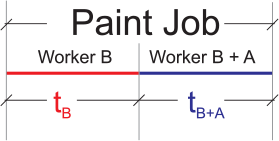# Star Problem: Work Rate – Painting Job## Problem:

A worker can finish the painting job in 15 days. Another worker can finish only 75% of that job for the same time. At first, the second worker painted for several days and then the first worker joined him and together they finished the rest of the work in 6 days. How many days each worker worked and what percent of the work has been done by each one of them.

### Given:

Worker A finishes a paint job in 15 days

Worker B finishes only 75% of the paint job in 15 daysa. Number of days each worker worked?

b. Percent of work done by each worker?

### Solution:

LET:   W – amount of work done; r – rate of work; t – duration of work

We know that, W = r  x  t     Eq. 1

Plotting the given data, we have:

 W r t Worker A (WA) 1 15 days Worker B (WB) 75% or 3/4 15 days

Using Eq. 1, we get:  r =  W  /  t

Rate of WA (rA)  =  1  /  15  =  1/15 per day

Rate of WB  (rB)  =  ¾  /  15  =  1/20 per day

Total work done per day of WA & WB = rA  +  r=  1/15  +  1/20  =  7/60 per day

Work done by WA & WB in 6 days = rA+B  x  number of days  =  7/60  x  6 days  =  7/10Portion of the paint job that WB worked alone = 1 – WA+B in 6 days = 1 – 7/10  =  3/10

Number of days WB worked alone = WB worked alone  /  Rate of WB  =  3/10  /  1/20  =  6 days

To solved for what is asked on (A), we get :

Number of days Worker A (WA) worked = 6 days  Ans.

Number of days Worker B (WB) worked = 6 days  +  6 days  =  12 days  Ans.

To solved for percent of work done by each worker (B):

 W r t Worker A (WA) 1/15 per day 6 days Worker B (WB) 1/20 per day 12 days

Work done of WA = rA  x  t=  1/15  x  6 days  =  2/5 or 40%  Ans.

Work done of WB = rB  x  tB  =  1/20  x  12 days  =  3/5 or 60%  Ans.